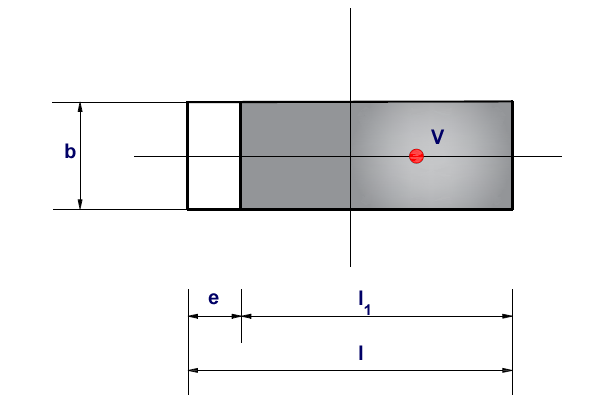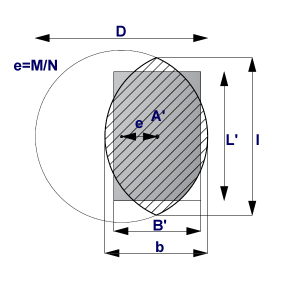The vertical bearing capacity of the foundation soil is verified according to the theory of limit states using the following inequality:

σ ≤ RdRV

or based on the factor of safety as:

Rd/σ ≥ SFv

where:

σ-extreme design contact stress at the footing bottom
Rd-design bearing capacity of foundation soil
γRV-coefficient of vertical bearing capacity of foundation
SFv-safety factor for vertical bearing capacity

Extreme design contact stress at the footing bottom assumed the form:

σ=V/Aef

where:

V-extreme design vertical force

Aef-effective area of foundation

Effective Area

When solving the problem of eccentrically loaded foundations the program offers two options to deal with an effective dimension of the foundation area:

• a rectangular shape of effective area is assumed
• circular shape of effective area is assumed

Rectangular shape
A simplified solution is used in such cases. In case of axial eccentricity (bending moment acts in one plane only) the analysis assumes a uniform distribution of contact stress σ applied only over a portion of the foundation l1, which is less by twice the eccentricity e compared to the total length l.Determination of effective area in case of axial eccentricity

An effective area (b*l1) is assumed to compute the contact stress, so that we have:

σ=V/b(l-2e)

In case of a general eccentric load (foundation is loaded by the vertical force V and by bending moments M1 and M2) the load is replaced by a single force with given eccentricities:

e1=M1/V

e2=M2/V

The size of effective area follows from the condition that the force V must act eccentrically:

Aef=bef ·lef = (b-2e2)(l-2e1)

Circular shape
A circular foundation subjected to a vertical load applied with an eccentricity e=Md/Nd can be regarded as an equivalent fictitious foundation with a centrally applied load (figure below), as suggested by Meyerhof (1953) and Vesic (1973). In this case, the area of the fictitious foundation, A’, can be calculated as:

A’=D2/2(arccos2e/D-2eD[1-(2e/D)2]0.5)

The aspect ratio of the equivalent rectangular area can also be approximated as the ratio of the line lengths b to l, as shown in the figure below that is,

B’/L’=b/l=[(D-2e)/(D+2e)]0.5Equivalent uniformly loaded rectangular area for eccentrically loaded circular foundation

LoadCap by GeoStru – Software for the computation of bearing capacity on loose or rocky soils

Supported computation standards:
• Eurocode 7/8
• British Codes BS8004
• SR EN 1997-1/NB/2007
• Other standards